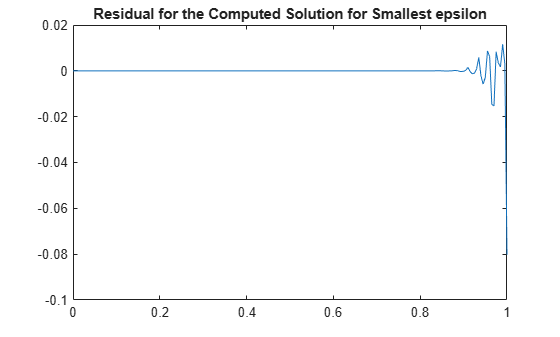Documentation

### This is machine translation

Mouseover text to see original. Click the button below to return to the English version of the page.

## Solving a Nonlinear ODE with a Boundary Layer by Collocation

This example shows how to use spline commands from Curve Fitting Toolbox™ solve a nonlinear ordinary differential equation (ODE).

### The Problem

We consider the nonlinear singularly perturbed problem

` epsilon D^2g(x) + (g(x))^2 = 1 on [0..1]`

` Dg(0) = g(1) = 0.`

This problem is already quite difficult for `epsilon = .001`, so we choose a modest

`epsilon = .1;`

### The Approximation Space

We seek an approximate solution by collocation from C^1 piecewise cubics with a specified break sequence `breaks`, hence want the order `k` to be 4.

```breaks = (0:4)/4; k = 4;```

We obtain the corresponding knot sequence as

`knots = augknt(breaks,k,2)`
```knots = 1×14 0 0 0 0 0.2500 0.2500 0.5000 0.5000 0.7500 0.7500 1.0000 1.0000 1.0000 1.0000 ```

Whatever the choice of order and knots, the corresponding spline space has dimension

`n = length(knots) - k`
```n = 10 ```

### Discretization

The number of degrees of freedom, 10, fits nicely with the fact that we expect to collocate at two sites per polynomial piece, for a total of 8 conditions, bringing us to 10 conditions altogether once we add the two side conditions.

We choose two Gauss sites for each interval. For the `standard' interval [-1/2 .. 1/2] of unit length, these are the two sites

`gauss = .5773502692*[-1/2; 1/2];`

From this, we obtain the whole collection of collocation sites by

```ninterv = length(breaks)-1; temp = (breaks(2:ninterv+1)+breaks(1:ninterv))/2; temp = temp([1 1],:) + gauss*diff(breaks); colsites = temp(:).';```

### The Numerical Problem

The numerical problem we want to solve is to find a piecewise polynomial (or pp) `y` of the given order, and with the given knots, that satisfies the nonlinear system

` Dy(0) = 0`

` (y(x))^2 + epsilon D^2y(x) = 1 for x in colsites`

` y(1) = 0`

### Linearization

If `y` is our current approximation to the solution, then the linear problem for the better (?) solution `z` by Newton's method reads

` Dz(0) = 0`

` w_0(x)z(x) + epsilon D^2z(x) = b(x) for x in colsites`

` z(1) = 0`

with `w_0(x) := 2y(x)` and `b(x) := (y(x))^2 + 1`.

In fact, by choosing `w_0(1) := 1`, `w_1(0) := 1`, and

` w_2(x) := epsilon, w_1(x) := 0 for x in colsites`

and choosing all other values of `w_0`, `w_1`, `w_2`, and `b` not yet specified to be zero, we can give our system the uniform shape

` w_0(x)z(x) + w_1(x)Dz(x) + w_2(x)D^2z(x) = b(x) for x in sites`

where

`sites = [0,colsites,1];`

### Linear System to be Solved

This system converts into one for the B-spline coefficients of its solution `z`. For this, we need the zeroth, first, and second derivative at every `x` in `sites` and for every relevant B-spline. These values are supplied by the `spcol` command.

Here is the essential part of the documentation for `spcol`:

`SPCOL B-spline collocation matrix.`

` COLLOC = SPCOL(KNOTS,K,TAU) is the matrix`

` [ D^m(i)B_j(TAU(i)) : i=1:length(TAU), j=1:length(KNOTS)-K ],`

` with D^m(i)B_j the m(i)-fold derivative of B_j,`

` B_j the j-th B-spline of order K for the knot sequence KNOTS,`

` TAU a sequence of sites,`

` both KNOTS and TAU are assumed to be nondecreasing, and`

` m(i) is the integer #{ j<i : TAU(j) = TAU(i) }, i.e., the`

` 'cumulative' multiplicity of TAU(i) in TAU.`

We use `spcol` to supply the matrix

`colmat = spcol(knots,k, brk2knt(sites,3));`

with `brk2knt` used here to triple each entry of `sites`, and thus we get in `colmat`, for each `x` in `sites`, the value and the first and second derivatives at `x` of all the relevant B-splines.

From this, we get the collocation matrix by combining the row triple associated with `x` using the weights `w_0(x), w_1(x), w_2(x)` to get the row corresponding to `x` of the matrix of our linear system.

### Need Initial Guess for Y

We also need a current approximation `y` from our spline space. Initially, we get it by interpolating some reasonable initial guess from our pp space at `sites`. For that guess, we use the parabola

` x^2 - 1`

which does satisfy the end conditions and lies in our spline space. We obtain its B-form by interpolation at `sites`. We select the relevant interpolation matrix from the full matrix `colmat`. Here it is, in several cautious steps:

```intmat = colmat([2 1+(1:(n-2))*3,1+(n-1)*3],:); coefs = intmat\[0 colsites.*colsites-1 0].'; y = spmak(knots,coefs.');```

We plot the result, to be sure -- it should be exactly `x^2-1`.

```fnplt(y,'g'); legend('Initial Guess (x^2-1)','location','NW'); axis([-0.01 1.01 -1.01 0.01]); hold on```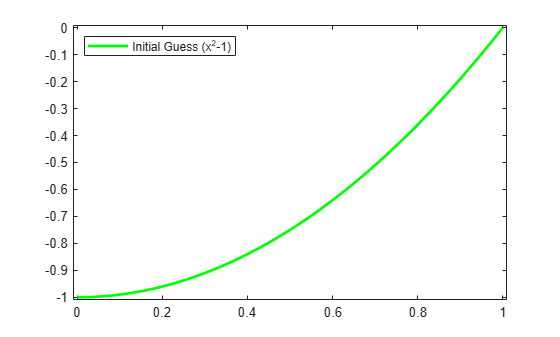### Iteration

We can now complete the construction and solution of the linear system for the improved approximate solution `z` from our current guess `y`. In fact, with the initial guess `y` available, we now set up an iteration, to be terminated when the change `z-y` is less than a specified tolerance.

`tolerance = 6.e-9;`

The max-norms of the change `z-y` at each iteration are shown as output below, and the figure shows each of the iterates.

```while 1 vtau = fnval(y,colsites); weights = [0 1 0; [2*vtau.' zeros(n-2,1) repmat(epsilon,n-2,1)]; 1 0 0]; colloc = zeros(n,n); for j = 1:n colloc(j,:) = weights(j,:)*colmat(3*(j-1)+(1:3),:); end coefs = colloc\[0 vtau.*vtau+1 0].'; z = spmak(knots,coefs.'); fnplt(z,'k'); maxdif = max(max(abs(z.coefs-y.coefs))); fprintf('maxdif = %g\n',maxdif) if (maxdif<tolerance), break, end % now reiterate y = z; end```
```maxdif = 0.206695 ```
```maxdif = 0.01207 ```
```maxdif = 3.95151e-05 ```
```maxdif = 4.43216e-10 ```
`legend({'Initial Guess (x^2-1)' 'Iterates'},'location','NW');`That looks like quadratic convergence, as expected from a Newton iteration.

### Getting Ready for a Smaller Epsilon

If we now decrease `epsilon`, we create more of a boundary layer near the right endpoint, and this calls for a nonuniform mesh. We use `newknt` to construct an appropriate (finer) mesh from the current approximation.

```knots = newknt(z, ninterv+1); breaks = knt2brk(knots); knots = augknt(breaks,4,2); n = length(knots)-k;```

### Collocation Sites for New Breaks

Next, we get the collocation sites corresponding to the new `breaks`

```ninterv = length(breaks)-1; temp = ((breaks(2:ninterv+1)+breaks(1:ninterv))/2); temp = temp([1 1], :) + gauss*diff(breaks); colsites = temp(:).'; sites = [0,colsites,1];```

and then the new collocation matrix.

`colmat = spcol(knots,k, brk2knt(sites,3));`

### Initial Guess

We obtain the initial guess `y` as the interpolant from the current spline space to the computed solution `z`. We plot the resulting interpolant to be sure -- it should be close to our current solution.

```intmat = colmat([2 1+(1:(n-2))*3,1+(n-1)*3],:); y = spmak(knots,[0 fnval(z,colsites) 0]/intmat.'); fnplt(y,'c'); ax = gca; h = ax.Children; legend(h([6 5 1]),{'Initial Guess (x^2-1)' 'Iterates' ... 'New Initial Guess for New Value of epsilon'}, ... 'location','NW');```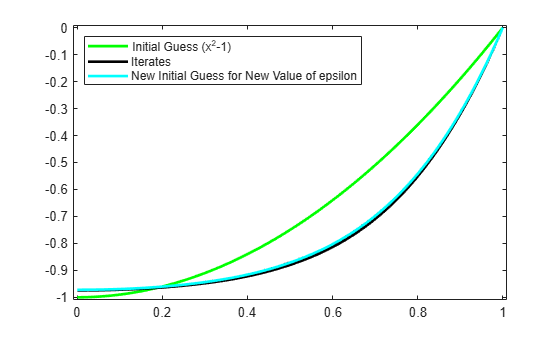### Iteration with Smaller Epsilon

Now we divide `epsilon` by 3 and repeat the above iteration. Convergence is again quadratic.

```epsilon = epsilon/3; while 1 vtau = fnval(y,colsites); weights = [0 1 0; [2*vtau.' zeros(n-2,1) repmat(epsilon,n-2,1)]; 1 0 0]; colloc = zeros(n,n); for j = 1:n colloc(j,:) = weights(j,:)*colmat(3*(j-1)+(1:3),:); end coefs = colloc\[0 vtau.*vtau+1 0].'; z = spmak(knots,coefs.'); fnplt(z,'b'); maxdif = max(max(abs(z.coefs-y.coefs))); fprintf('maxdif = %g\n',maxdif) if (maxdif<tolerance), break, end % now reiterate y = z; end```
```maxdif = 0.237937 ```
```maxdif = 0.0184488 ```
```maxdif = 0.000120467 ```
```maxdif = 4.78115e-09 ```
```ax = gca; h = ax.Children; legend(h([10 9 5 4]), ... {'Initial Guess (x^2-1) for epsilon = .1' 'Iterates' ... sprintf('Initial Guess for epsilon = %.3f',epsilon) ... 'Iterates'}, 'location','NW');```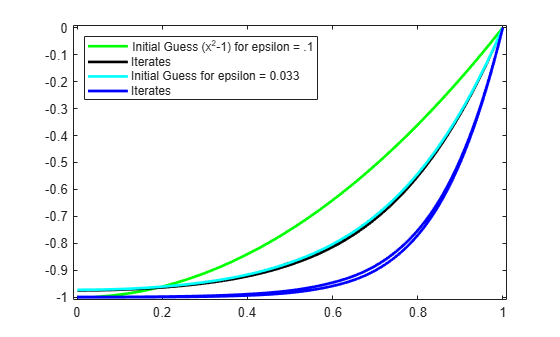### Very Small Epsilon

For a much smaller `epsilon`, we merely repeat these calculations, dividing `epsilon` by 3 each time.

```for ee = 1:4 knots = newknt(z, ninterv+1); breaks = knt2brk(knots); knots = augknt(breaks,4,2); n = length(knots)-k; ninterv = length(breaks)-1; temp = ((breaks(2:ninterv+1)+breaks(1:ninterv))/2); temp = temp([1 1], :) + gauss*diff(breaks); colsites = temp(:).'; sites = [0,colsites,1]; colmat = spcol(knots,k, brk2knt(sites,3)); intmat = colmat([2 1+(1:(n-2))*3,1+(n-1)*3],:); y = spmak(knots,[0 fnval(z,colsites) 0]/intmat.'); fnplt(y,'c') epsilon = epsilon/3; while 1 vtau = fnval(y,colsites); weights = [0 1 0; [2*vtau.' zeros(n-2,1) repmat(epsilon,n-2,1)]; 1 0 0]; colloc = zeros(n,n); for j = 1:n colloc(j,:) = weights(j,:)*colmat(3*(j-1)+(1:3),:); end coefs = colloc\[0 vtau.*vtau+1 0].'; z = spmak(knots,coefs.'); fnplt(z,'b'); maxdif = max(max(abs(z.coefs-y.coefs))); if (maxdif<tolerance), break, end % now reiterate y = z; end end ax = gca; h = ax.Children; legend(h([30 29 25 24]), ... {'Initial Guess (x^2-1) for epsilon = .1' 'Iterates' ... 'Initial Guesses for epsilon = .1/3^j, j=1:5' ... 'Iterates'},'location','NW');```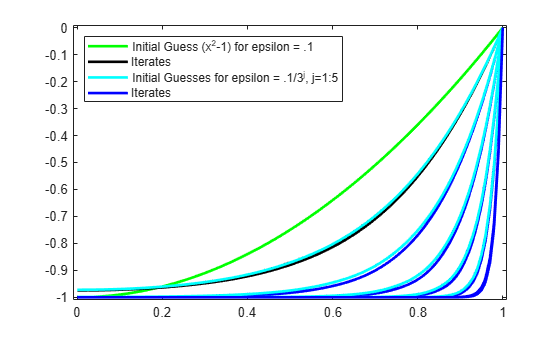### Plot the Breaks Used for Smallest Epsilon

Here is the final distribution of `breaks`, showing `newknt` to have worked well in this case.

```breaks = fnbrk(fn2fm(z,'pp'),'b'); bb = repmat(breaks,3,1); cc = repmat([0;-1;NaN],1,length(breaks)); plot(bb(:),cc(:),'r'); hold off ax = gca; h = ax.Children; legend(h([31 30 26 25 1]), ... {'Initial Guess (x^2-1) for epsilon = .1' 'Iterates' ... 'Initial Guesses for epsilon = .1/3^j, j=1:5' ... 'Iterates' 'Breaks for epsilon = .1/3^5'},'location','NW');```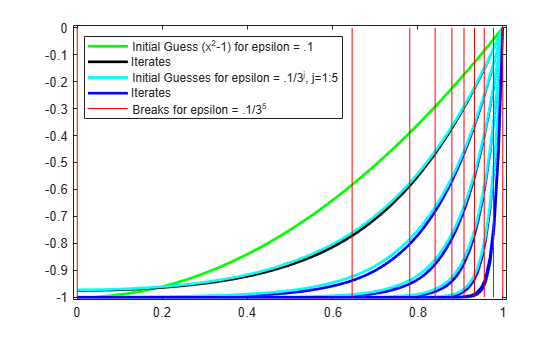### Plot Residual for Smallest Epsilon

Recall that we are solving the ODE

` epsilon D^2g(x) + (g(x))^2 = 1 on [0..1]`

As a check, we compute and plot the residual for the computed solution for the smallest epsilon. This, too, looks satisfactory.

```xx = linspace(0,1,201); plot(xx, 1 - epsilon*fnval(fnder(z,2),xx) - (fnval(z,xx)).^2) title('Residual for the Computed Solution for Smallest epsilon');```